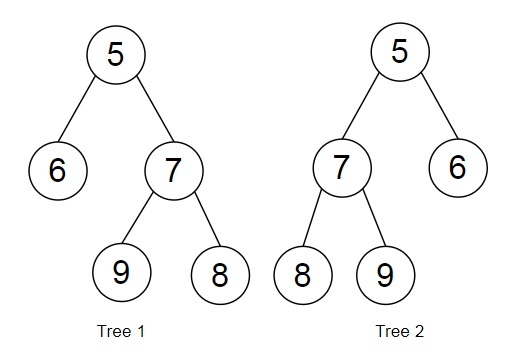# Check if all levels of two trees are anagrams or not in Python

Suppose, we are provided with two binary trees. We have to check if each level of a binary tree is an anagram of the other binary tree's same level. We return True if it is an anagram, otherwise we return False.

So, if the input is like, then the output will be True.

To solve this, we will follow these steps −

• tree_1 is the root node of the first tree and tree_2 is the root node of the second tree.
• if tree_1 is same as null and tree_2 is same as null, then
• return True
• if tree_1 is same as null or tree_2 is same as null, then
• return False
• queue1 := a new queue
• queue2 := a new queue
• insert tree_1 at the end of queue1
• insert tree_2 at the end of queue2
• while 1 is non-zero, do
• size1 := size of queue1
• size2 := size of queue2
• if size1 is not same as size2, then
• return False
• if size1 is same as 0, then
• come out from the loop
• curr_level1 := a new list
• curr_level2 := a new list
• while size1 > 0, do
• node1 := first element of queue1
• delete first element from queue1
• if left of node1 is not same as null, then
• insert left of node1 at the end of queue1
• if right of node1 is not same as null, then
• insert right of node1 at the end of queue1
• size1 := size1 - 1
• node2 := first element of queue2
• delete first element from queue2
• if left of node2 is not same as null, then
• insert left of node2 at the end of queue2
• if right of node2 is not same as null, then
• insert right of node2 at the end of queue2
• insert value of node1 at the end of curr_level1
• insert value of node2 at the end of curr_level2
• sort the list curr_level1
• sort the list curr_level2
• if curr_level1 is not same as curr_level2, then
• return False
• return True

## Example

Let us see the following implementation to get better understanding −

Live Demo

def make_tree(elements):
tree = tree_node(elements)
for element in elements[1:]:
insert_value(tree, element)
return tree
def insert_value(temp,value):
que = []
que.append(temp)
while (len(que)):
temp = que
que.pop(0)
if (not temp.left):
if value is not None:
temp.left = tree_node(value)
else:
temp.left = tree_node(0)
break
else:
que.append(temp.left)
if (not temp.right):
if value is not None:
temp.right = tree_node(value)
else:
temp.right = tree_node(0)
break
else:
que.append(temp.right)
class tree_node:
def __init__(self, value):
self.value = value
self.left = None
self.right = None
def solve(tree_1, tree_2) :
if (tree_1 == None and tree_2 == None) :
return True
if (tree_1 == None or tree_2 == None) :
return False
queue1 = []
queue2 = []
queue1.append(tree_1)
queue2.append(tree_2)
while (1) :
size1 = len(queue1)
size2 = len(queue2)
if (size1 != size2):
return False
if (size1 == 0):
break
curr_level1 = []
curr_level2 = []
while (size1 > 0):
node1 = queue1
queue1.pop(0)
if (node1.left != None) :
queue1.append(node1.left)
if (node1.right != None) :
queue1.append(node1.right)
size1 -= 1
node2 = queue2
queue2.pop(0)
if (node2.left != None) :
queue2.append(node2.left)
if (node2.right != None) :
queue2.append(node2.right)
curr_level1.append(node1.value)
curr_level2.append(node2.value)
curr_level1.sort()
curr_level2.sort()
if (curr_level1 != curr_level2) :
return False
return True
tree_1 = make_tree([5, 6, 7, 9, 8])
tree_2 = make_tree([5, 7, 6, 8, 9])
print(solve(tree_1, tree_2))

## Input

[5, 6, 7, 9, 8], [5, 7, 6, 8, 9]

## Output

True